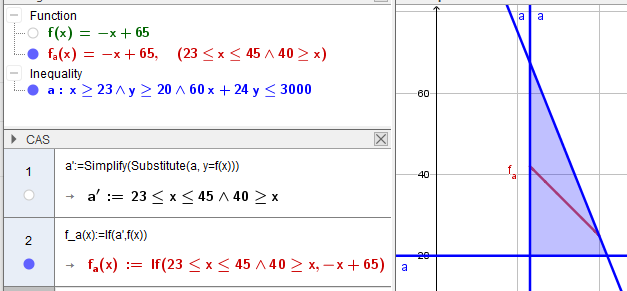# Intersection between a group of inequalities and an equation.

Abel Mendez shared this question 2 years ago

Hello, I thank in advance the people who can help me in this matter:

I am working on solving linear programming problems and I have problems graphing them when among the constraints an equality is included. For example:

I have the following constraints:

x≥23

y≥20

60 x+24 y≤3000

x+y=65

When I want to graph I place the following: x≥23 ∧ y≥20 ∧ 60 x+24 y≤3000 ∧ x+y=65; but I get an error. I understand that since there is no "feasible area" in the problem it does not graph it; however the intersection of these constraints would be a segment (see red line in the image).

Is there any way to visually obtain the segment in the application as well as the start and end coordinates or to check if the line crosses the area formed by the inequations (similar to IsInRegion but with lines).1

I don't think there is a simple, "native" solution in Geogebra, so you have to tell Geogebra how to construct that segment. Attached is a way to do it. It should be able to handle any number of linear inequalities with a single linear equality, as long as the final intersection is bounded (i.e. no whole line or ray).

I couldn't find a way to "deconstruct" the region and get the inequalities back to work with those, so as a starting point it's assumed that the system of inequalities is given as a standardized matrix-vector inequality (Ax<=b). The matrix A and vector b should have proper sizes (I didn't include any checks for that).

The region satisfying the inequality is then constructed from the matrix A and the vector b. (It's a bit complicated via an auxiliary list, but I couldn't find an easy way to do it.)

To get the the intersection of that region with the line that represents the given equality, first the intersections of that line with the boundary lines of each inequality is computed. (Geogebra only gives a proper result when the lines are not parallel, but only those are needed anyway.)

The resulting list of points is then trimmed, keeping only the points that are in the inequality region, and each of those points only once. Because the list can only contain points on the boundary of the region and because the region is convex, this means that the list contains at most two points in the end. If two then those are the endpoints of the segment; if one then it's just assumed that the interersection is a single corner point (I didn't handle the case of an infinite region where the intersection might be a whole ray); if none then the intersection is assumed to be empty (I didn't handle the case of an infinite region where the intersection might be the whole line).

Then the segment (if it exists) is drawn, and some text output is generated.1

The Vertex() command can be helpful in some cases.1

Ah, nice. I didn't know that Vertex command. Much easier that way.1

In other cases the command ClosestPointRegion()1

Und noch einen....

an equation given as f(x)=... and with CAS part11

Thank you very much for your amazing support, I see that there are several ways to achieve the result. I'll be testing them all and see which one fits my use case the best. Thank you again